The resistance of a wire is 10–6 Ω per metre. It is bend in the form of a circle of diameter 2 m. A wire of the same material is connected across its diameter. The total resistance across its diameter AB will be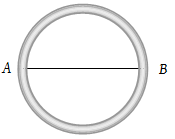(1) $\frac{4}{3}\pi ×{10}^{-6}\Omega$

(2) $\frac{2}{3}\pi ×{10}^{-6}\Omega$

(3) $0.88×{10}^{-6}\Omega$

(4) $14\pi ×{10}^{-6}\Omega$

Concept Questions :-

Combination of resistors
High Yielding Test Series + Question Bank - NEET 2020

Difficulty Level:

In the figure shown, the capacity of the condenser C is $2\text{\hspace{0.17em}}\mu F$. The current in 2 Ω resistor is :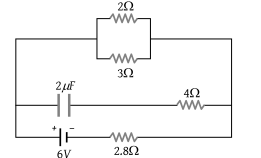(1) 9 A

(2) 0.9 A

(3) $\frac{1}{9}A$

(4) $\frac{1}{0.9}A$

Concept Questions :-

Kirchoff's voltage law
High Yielding Test Series + Question Bank - NEET 2020

Difficulty Level:

When the key K is pressed at time t = 0, which of the following statements about the current I in the resistor AB of the given circuit is true?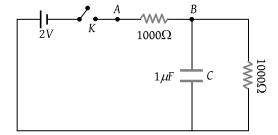(1) I = 2 mA at all t

(2) I oscillate between 1 mA and 2mA

(3) I = 1 mA at all t

(4) At t = 0 , I = 2 mA and with time it goes to 1 mA

Concept Questions :-

Kirchoff's voltage law
High Yielding Test Series + Question Bank - NEET 2020

Difficulty Level:

A torch bulb rated as 4.5 W, 1.5 V is connected as shown in the figure. The e.m.f. of the cell needed to make the bulb glow at full intensity is :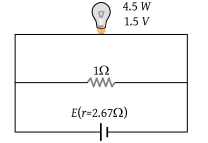(1) 4.5 V

(2) 1.5 V

(3) 2.67 V

(4) 13.5 V

Concept Questions :-

Heating effect of current
High Yielding Test Series + Question Bank - NEET 2020

Difficulty Level:

In the circuit shown in the figure, the current through :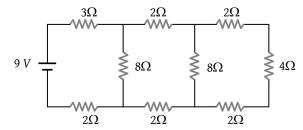1. The 3Ω resistor is 0.50A

2. The 3Ω resistor is 0.25 A

3. The 4Ω resistor is 0.50A

4. The 4Ω resistor is 0.25 A

Concept Questions :-

Combination of resistors
High Yielding Test Series + Question Bank - NEET 2020

Difficulty Level:

There are three resistance coils of equal resistance. The maximum number of resistances you can obtain by connecting them in any manner you choose, being free to use any number of the coils in any way is :

(1) 3

(2) 4

(3) 6

(4) 5

Concept Questions :-

Combination of resistors
High Yielding Test Series + Question Bank - NEET 2020

Difficulty Level:

If in the circuit shown below, the internal resistance of the battery is 1.5 Ω and VP and VQ are the potentials at P and Q respectively, what is the potential difference between the points P and Q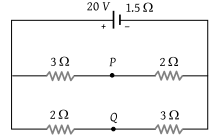(1) Zero

(2) 4 volts (VP > VQ)

(3) 4 volts (VQ > VP)

(4) 2.5 volts (VQ > VP)

Concept Questions :-

Kirchoff's voltage law
High Yielding Test Series + Question Bank - NEET 2020

Difficulty Level:

Two wires of resistance R1 and R2 have temperature coefficient of resistance , respectively. These are joined in series. The effective temperature coefficient of resistance is :

(1) $\frac{{\alpha }_{1}+{\alpha }_{2}}{2}$

(2) $\sqrt{{\alpha }_{1}{\alpha }_{2}}$

(3) $\frac{{\alpha }_{1}{R}_{1}+{\alpha }_{2}{R}_{2}}{{R}_{1}+{R}_{2}}$

(4) $\frac{\sqrt{{R}_{1}{R}_{2}{\alpha }_{1}{\alpha }_{2}}}{\sqrt{{R}_{1}^{2}+{R}_{2}^{2}}}$

Concept Questions :-

Combination of resistors
High Yielding Test Series + Question Bank - NEET 2020

Difficulty Level:

Two cells of equal e.m.f. and of internal resistances, r1, and ${r}_{2}\left({r}_{1}>{r}_{2}\right)$ are connected in series. On connecting this combination to an external resistance R, it is observed that the potential difference across the first cell becomes zero. The value of R will be :

(1) ${r}_{1}+{r}_{2}$

(2) ${r}_{1}-{r}_{2}$

(3) $\frac{{r}_{1}+{r}_{2}}{2}$

(4) $\frac{{r}_{1}-{r}_{2}}{2}$

Concept Questions :-

Grouping of cells
High Yielding Test Series + Question Bank - NEET 2020

Difficulty Level:

When connected across the terminals of a cell, a voltmeter measures 5V and a connected ammeter measures 10 A of current. A resistance of 2 ohms is connected across the terminals of the cell. The current flowing through this resistance will be :

(1) 2.5 A

(2) 2.0 A

(3) 5.0 A

(4) 7.5 A

Concept Questions :-

Kirchoff's voltage law